# An easy guide for P-Value calculation and applicationsPosted by

#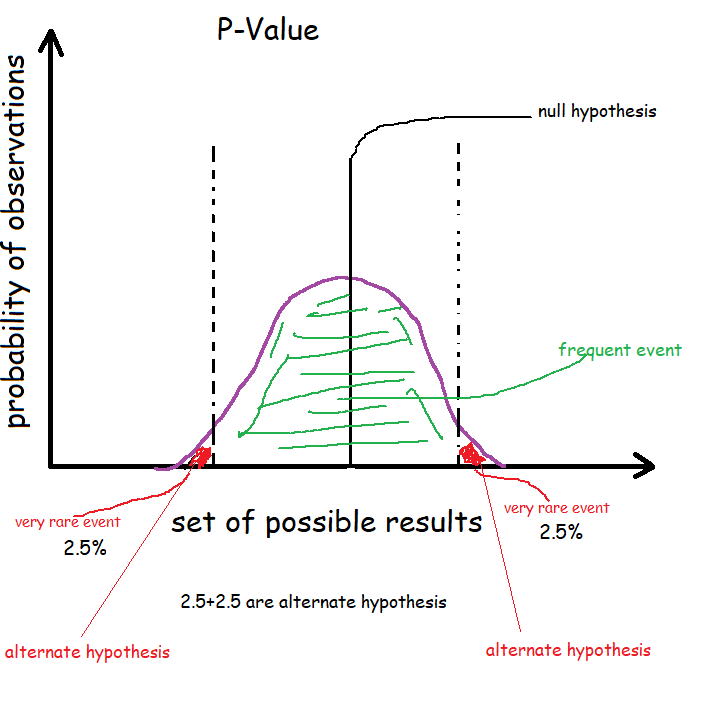## Outline:

• Introduction
• What is p-value
• How p value is calculated
• P value in  feature selection
• Get P value using python
• Conclusion

## Introduction

Whenever we want to prove some statement(hypothesis) or if we won’t prove it wrong, there has to be some measure.  Generally, we give an argument in favor of or in against a hypothesis. P-value is a term in the statistic that decides whether to accept or to reject the hypothesis.

## What is p-value?

Definition: a p-value is a probability that random chance generated a value or something else that is equal or rear. In other words, it is the probability of observing a value or effect equivalent to a value or effect observed when the null hypothesis is true.

## How to calculate P-value?

### Example 1 for the categorical problem:

Let’s assume we have a coin and we are flipping it two times. What will be the probability of getting two heads consecutively and what will be the p-value if we get two heads in a row?

So, if we flip a coin for the first time, there is a 50% probability we shall get head, similarly, there is a 50% probability we shall get tail. Now, the same story goes with the second flip.### Probability of getting two heads:

if we flip a coin for the first time, there is a 50% chance that we shall get head and a 50% chance for getting tail. Now for the second event also, if we flip a coin there is a 50% chance that we shall get head and a 50% chance of getting tail. The image below shows how it is done.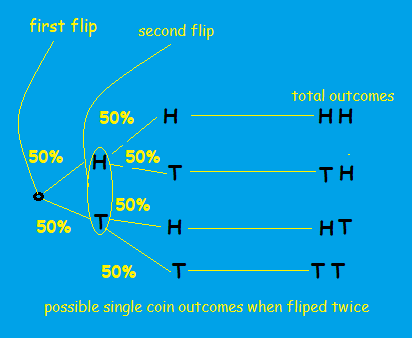Therefore the probability of getting two heads consecutively is:Similarly, the probability of getting two tails consecutively is:The probability of two tails is considered because, for calculating the P-value of an event, we have to consider the probability of the target event(probability of heads= 0.25) + the probability of an equally

opposite event(getting two tails in this case=0.25)+ probability of some event that is more rear(0).

The P-value of getting two heads consecutively is 0.25+0.25+0=0.5 or 50%.

### Example 2, calculating p-value of the continuous variable:

Let assume, we have a bucket of apples, and these apples measure between 180grams to 120grams and we want to know what will be p-value of an apple that weighs 140 grams?

Well, for a problem like these we use a density/distribution graph.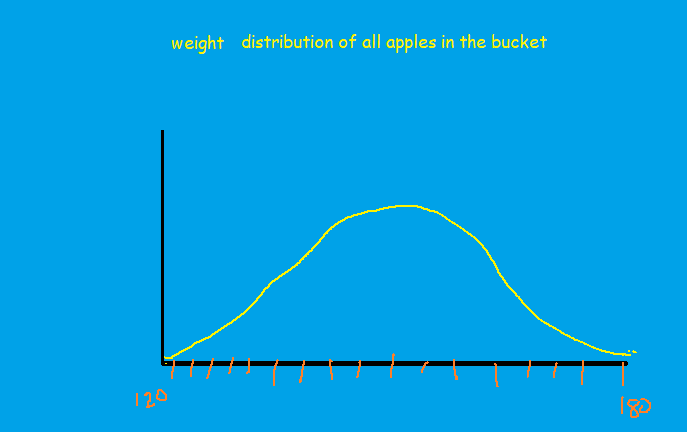The area under the curve is the probability of the weight of apples. In data science, this can be the distribution of a feature for which we want to check the P-values.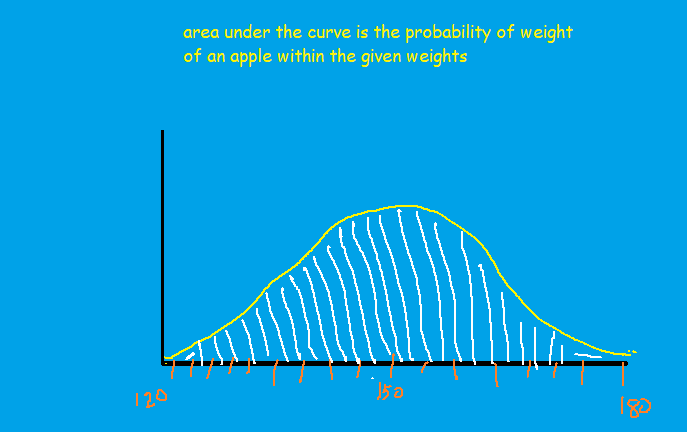From the distribution, we can infer that 95% of the apples weigh between 140 to 170 grams. Therefore it indicates most of the apples are between 140 to 170grams. In other words, if we take out an apple randomly, there will be a 95% chance that it will be between 140 to 170 grams.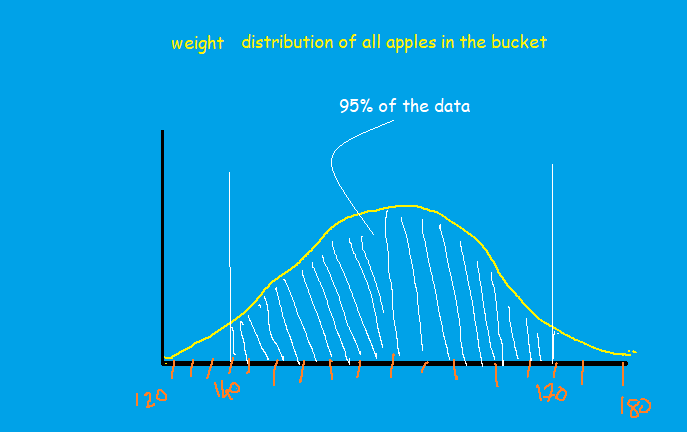2.5% of the data under the curve is greater than 170grams. In other words, if we take out an apple from the bucket there will be a 2.5% probability of getting an apple heavier than 170 grams(image_7)

Similarly, there is a 2.5% probability of getting an apple that is lighter than 140 grams(image_7).Now to calculate the p-value of an apple that weighs 140grams, we shall add 2.5% of apples that are less than 140 grams and 2.5% of apples that are more than 170 grams, which becomes 5%.

Let’s revise how to calculate value again, it is the probability that random chance generated a value or something else that is equal or rearer.

So, 2.5% less than 140 gram(this is the event of interest) + 2.5% more than 170grams(opposite of the event of interest) + 0(something that is more rearer)

### Example 3, what will be the p-value of an apple that weighs between 155gram to 156grams?The area between 155 and 156 can be seen as a thick line whose area 1*1 cm square(4%), which is very less. So, to calculate P-value, in this case, will be, area of the line + 48% of the area those apples that are less than 155 + 48% of the area that are more than 156. Which results in 100%.Therefore, any data point or any region that is very very close to the mean of the distribution, p-value becomes high or 100%. Which indicates there is nothing unusual in the distribution.

## Feature selection using P-value:

One of the important use of P-value in machine learning is feature selection. Each feature in the dataset has its own distribution graph. From the distribution graph, we can compare how much each features different from the other.

If the P-value of a feature is high this means the p-value is very near to the mean of the distribution, this also indicates that there is nothing unusual about the feature. Therefore the feature is very very similar to the distribution of the other features, which means it does not carry any new pieces of information. Hence, we can drop it.

Similarly, if the P-value is very small, then the distribution of the feature is different from the others. Therefore, that feature carries some more information that can be helpful for the target variable.

## Get P value using python

https://docs.scipy.org/doc/scipy/reference/generated/scipy.stats.ttest_ind.html

```from scipy import stats
import numpy as np
x1=np.random.randint(100, size=(5))
x2=np.random.randint(100, size=(5))
stats.ttest_ind(x1,x2)

```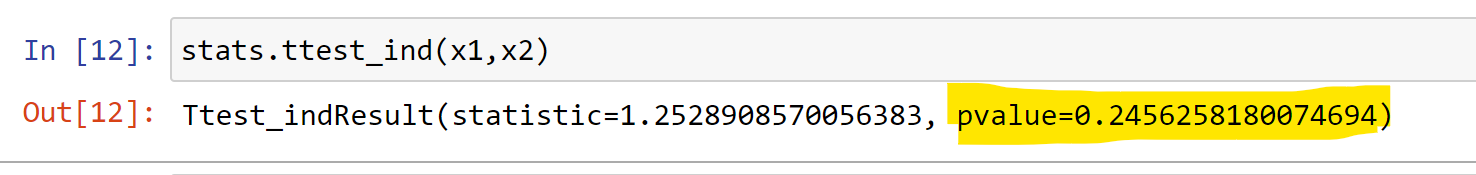```x3=np.random.randint(low=1000,high=10000, size=(5))
stats.ttest_ind(x1,x3)

```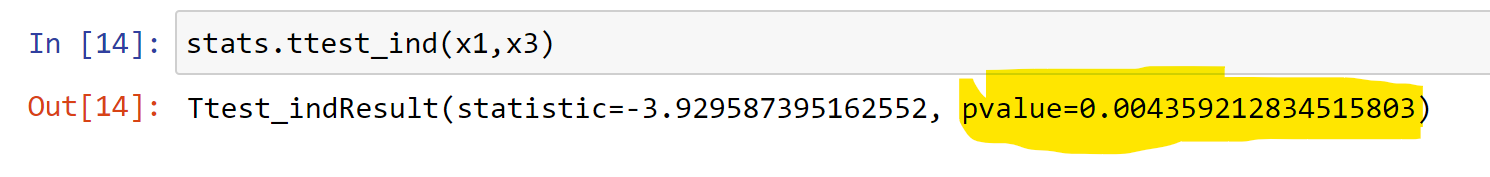If we observe carefully p-value in the first case is 0.24 and the p-value in the second case is 0.004. P-value in the first case is very small because both the distribution(x1 and x3) are different whereas the distribution of x1 and x2 is very similar.

## Important points to remember

•  The higher the p-value of a variable, it’s closer to an average of the target variable’s distribution.
•    The higher the p-value of a variable, its distribution is similar to the distribution of the target variable.
•   The lower p-value, this means, the variable belongs to some other distribution OR Some other distribution can explain the data better.
•  Null Hypothesis: there is nothing extra-ordinary
• Alternate Hypothesis: something else happening.
•  Null hypothesis wrt to data science: There no relation between a feature  & the target variable in Linear Regression.
• We have to reject or accept the Null Hypothesis using P-value.

## Conclusion

In this blog, we learn about p-value and how it is calculated for categorical and continuous features. We also learn how p-value can be used for Hypothesis testing and how it is used for feature selection. In our upcoming blog, we shall learn about ANOVA and Hypothesis testing.